# B: Extract each number present in the image

`import numpyimport cv2from keras.datasets import mnistfrom keras.models import Sequentialfrom keras.layers import Densefrom keras.layers import Dropoutfrom keras.layers import Flattenfrom keras.layers.convolutional import Conv2Dfrom keras.layers.convolutional import MaxPooling2Dfrom keras.utils import np_utilsfrom keras import backend as Kimport matplotlib.pyplot as plt`
`K.set_image_dim_ordering('th')seed = 7numpy.random.seed(seed)(X_train, y_train), (X_test, y_test) = mnist.load_data()`
`X_train = X_train.reshape(X_train.shape, 1, 28,                           28).astype('float32')X_test = X_test.reshape(X_test.shape, 1, 28,                           28).astype('float32') X_train = X_train / 255X_test = X_test / 255y_train = np_utils.to_categorical(y_train)y_test = np_utils.to_categorical(y_test)num_classes = y_test.shape`
`model = Sequential()model.add(Conv2D(32, (5, 5), input_shape=(1, 28, 28),          activation='relu'))model.add(MaxPooling2D(pool_size=(2, 2)))model.add(Conv2D(16, (3,          3), activation='relu'))model.add(MaxPooling2D(pool_size=(2, 2)))model.add(Dropout(0.2))model.add(Flatten())model.add(Dense(128, activation='relu'))model.add(Dense(64, activation='relu'))model.add(Dense(num_classes, activation='softmax'))`
`model.compile(loss='categorical_crossentropy', optimizer='adam',               metrics=['accuracy'])model.fit(X_train, y_train, validation_data=(X_test, y_test),               epochs=10, batch_size=200)scores = model.evaluate(X_test, y_test, verbose=0)print("Large CNN Error: %.2f%%" % (100-scores*100))`
`test_images = X_test[1:5]test_images = test_images.reshape(test_images.shape, 28, 28)print ("Test images shape: {}".format(test_images.shape))for i, test_image in enumerate(test_images, start=1):    org_image = test_image    test_image = test_image.reshape(1,1,28,28)    prediction = model.predict_classes(test_image, verbose=0)    print ("Predicted digit: {}".format(prediction))    plt.subplot(220+i)    plt.axis('off')    plt.title("Predicted digit: {}".format(prediction))    plt.imshow(org_image, cmap=plt.get_cmap('gray'))plt.show()`
`# serialize model to JSONmodel_json = model.to_json()with open("model.json", "w") as json_file:    json_file.write(model_json)# serialize weights to HDF5model.save_weights("model.h5")print("Saved model to disk")`
`json_file = open('model.json', 'r')loaded_model_json = json_file.read()json_file.close()loaded_model = model_from_json(loaded_model_json)loaded_model.load_weights("model.h5")`
`sudoku = cv2.resize(sudoku, (450,450))grid = np.zeros([9,9])for i in range(9):    for j in range(9):        image = sudoku[i*50:(i+1)*50,j*50:(j+1)*50]        if image.sum() > 25000:                grid[i][j] = identify_number(image)        else:            grid[i][j] = 0    grid =  grid.astype(int)`
`def identify_number(image):    image_resize = cv2.resize(image, (28,28))    # For plt.imshow    image_resize_2 = image_resize.reshape(1,1,28,28)    # For input to model.predict_classes#    cv2.imshow('number', image_test_1)    loaded_model_pred = loaded_model.predict_classes(image_resize_2                                                       , verbose = 0)    return loaded_model_pred`

# C: Compute the solution of the sudoku using Backtracking Algorithm

`def find_empty_location(arr,l):    for row in range(9):        for col in range(9):            if(arr[row][col]==0):                l=row                l=col                return True    return False`
`def used_in_row(arr,row,num):    for i in range(9):           if(arr[row][i] == num):              return True    return False`
`def used_in_col(arr,col,num):    for i in range(9):          if(arr[i][col] == num):              return True    return False`
`def used_in_box(arr,row,col,num):    for i in range(3):        for j in range(3):            if(arr[i+row][j+col] == num):                 return True     return False`
`def check_location_is_safe(arr,row,col,num):    return not used_in_row(arr,row,num) and            not used_in_col(arr,col,num) and            not used_in_box(arr,row - row%3,col - col%3,num)`
`def solve_sudoku(arr):    l=[0,0]     if(not find_empty_location(arr,l)):        return True     row=l    col=l     for num in range(1,10):         if(check_location_is_safe(arr,row,col,num)):             arr[row][col]=num             if(solve_sudoku(arr)):                 return True             # failure, unmake & try again             arr[row][col] = 0         return False`
`def print_grid(arr):    for i in range(9):        for j in range(9):               print (arr[i][j])          print ('\n')`
`def sudoku_solver(grid):    if(solve_sudoku(grid)):        print('---')    else:        print ("No solution exists")    grid = grid.astype(int)    return grid`

# Conclusion

--

--

--

## More from Aakash Jhawar

Software Engineer, Machine Learning

Love podcasts or audiobooks? Learn on the go with our new app.

## What is Text Summarization in NLP?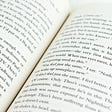## Machine-Learning powered Gesture Recognition on iOS## Sentiment Analysis on Movie Reviews with NLP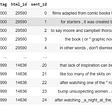## Machine Learning From scratch | Part 2. Vector Dot Product## Clustering## Release Machine Learning models as a service on Oracle Autonomous DB## Shallow Neural Networks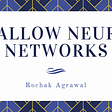## 【Paper Summary】 BRCNN for Relation Classification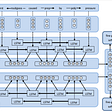## Aakash Jhawar

Software Engineer, Machine Learning

## Feedforward Neural Network (FNN) Implementation from Scratch Using Python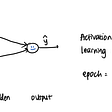## Cartoonize an Image using OpenCV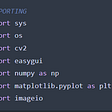## Deep Learning in Medicine: Identifying Pneumonia from a Chest-X-Ray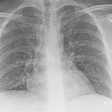## Creating gradient images using only NumPy